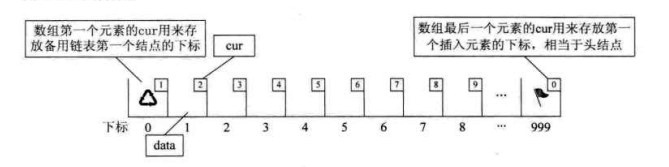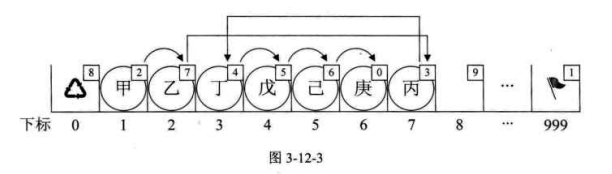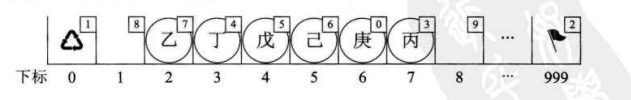1、初始化的时候除了分配一个数组的空间外，还需要做哪些操作？

1. 如何找到空闲的节点？

1. 如何知道静态链表是否已满？## 静态链表的插入操作

• 判断下标合法性
• 获取空闲节点的下标
• 写入数据到空闲节点
• 将上面空闲节点的 cur 修改为原 i 位置的 cur
• 将写入位置前一个节点的 cur 修改为新节点所在位置

• 当我们执行插入语句时，我们的目的是要在 “乙” 和 “丁” 之间插入 “丙”。调用代码时，输入 i 值为 3。
• 第 4 行让 k = MAX_SIZE - 1 = 999
• 第 7 行，j = Malloc_SLL(L) = 7。此时下标为 0 的 cur 也因为 7 要被占用而更改备用链表的值为 8。
• 第 11～12 行，for 循环 l 由 1 到 2，执行两次。代码 k = L[k].cur; 使得 k = 999，得到 k = L.cur = 1，再得到 k = L.cur = 2
• 第 13 行，L[j].cur = L[k].cur; 因 j=7，而 k = 2 得到 L.cur = L.cur = 3。这就是刚才说的 “丙” 把它的 cur 修改为 3 的意思。
• 第 14 行，L[k].cur = j; 意思就是 L.cur = 7。把它的 cur 改为指向 “丙” 的下标 7。## 静态链表的删除操作

L.cur = L.cur = 2。这其实就是告诉计算机现在 “甲” 已经离开了，”乙” 才是第一个元素。